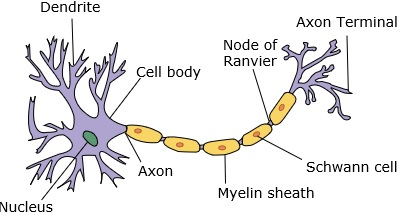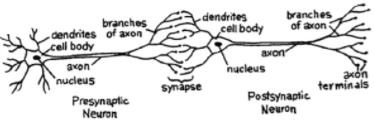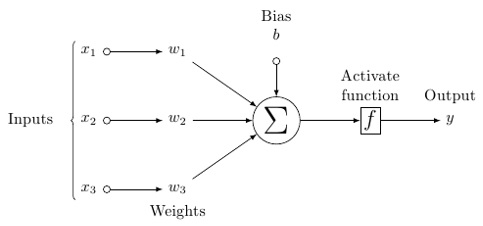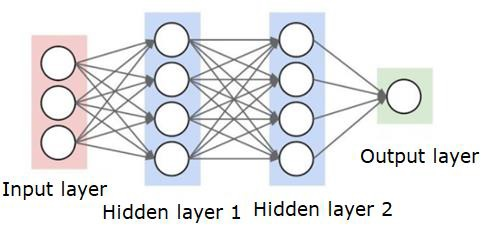# Artificial Neural Networks

The Artificial Neural Network, or just neural network for short, is not a new idea. It has been around for about 80 years.

It was not until 2011, when Deep Neural Networks became popular with the use of new techniques, huge dataset availability, and powerful computers.

A neural network mimics a neuron, which has dendrites, a nucleus, axon, and terminal axon.For a network, we need two neurons. These neurons transfer information via synapse between the dendrites of one and the terminal axon of another.A probable model of an artificial neuron looks like this −A neural network will look like as shown below −The circles are neurons or nodes, with their functions on the data and the lines/edges connecting them are the weights/information being passed along.

Each column is a layer. The first layer of your data is the input layer. Then, all the layers between the input layer and the output layer are the hidden layers.

If you have one or a few hidden layers, then you have a shallow neural network. If you have many hidden layers, then you have a deep neural network.

In this model, you have input data, you weight it, and pass it through the function in the neuron that is called threshold function or activation function.

Basically, it is the sum of all of the values after comparing it with a certain value. If you fire a signal, then the result is (1) out, or nothing is fired out, then (0). That is then weighted and passed along to the next neuron, and the same sort of function is run.

We can have a sigmoid (s-shape) function as the activation function.

As for the weights, they are just random to start, and they are unique per input into the node/neuron.

In a typical "feed forward", the most basic type of neural network, you have your information pass straight through the network you created, and you compare the output to what you hoped the output would have been using your sample data.

The act of sending data straight through a neural network is called a feed forward neural network.

Our data goes from input, to the layers, in order, then to the output.

When we go backwards and begin adjusting weights to minimize loss/cost, this is called back propagation.

This is an optimization problem. With the neural network, in real practice, we have to deal with hundreds of thousands of variables, or millions, or more.

The first solution was to use stochastic gradient descent as optimization method. Now, there are options like AdaGrad, Adam Optimizer and so on. Either way, this is a massive computational operation. That is why Neural Networks were mostly left on the shelf for over half a century. It was only very recently that we even had the power and architecture in our machines to even consider doing these operations, and the properly sized datasets to match.

For simple classification tasks, the neural network is relatively close in performance to other simple algorithms like K Nearest Neighbors. The real utility of neural networks is realized when we have much larger data, and much more complex questions, both of which outperform other machine learning models.